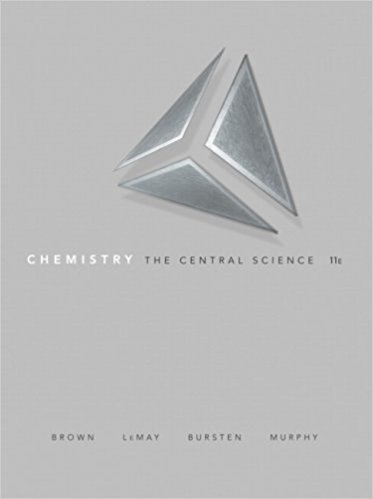×

# Fluorine has atomic number 9. If we represent the radius of a fluorine atom with the## Problem 7.2 Chapter 7

Chemistry: The Central Science | 11th Edition

• 2901 Step-by-step solutions solved by professors and subject experts
• Get 24/7 help from StudySoup virtual teaching assistantsChemistry: The Central Science | 11th Edition

4 5 0 316 Reviews
20
5
Problem 7.2

Fluorine has atomic number 9. If we represent the radius of a fluorine atom with the billiard ball illustrated here, would the analogy be more appropriate for the bonding or nonbonding atomic radius? If we used the same billiard ball to illustrate the concept of fluorine's bonding atomic radius, would we overestimate or underestimate the bonding atomic radius? Explain. [Section 7.3]

Step-by-Step Solution:
Step 1 of 3

Quiz 5 Chemical Equation: Shorthand way of specifying a chemical reaction. Reactants (left side) -> Products (right side) All chemical equations must be balanced: 1. Material balance: number of atoms of each element must be the same on both sides of the equation. 2. Charge Balance: net charge on reactant side must be equal to net charge...

Step 2 of 3

Step 3 of 3

#### Related chapters

Unlock Textbook Solution

Fluorine has atomic number 9. If we represent the radius of a fluorine atom with the

×
Get Full Access to Chemistry: The Central Science - 11 Edition - Chapter 7 - Problem 7.2

Get Full Access to Chemistry: The Central Science - 11 Edition - Chapter 7 - Problem 7.2